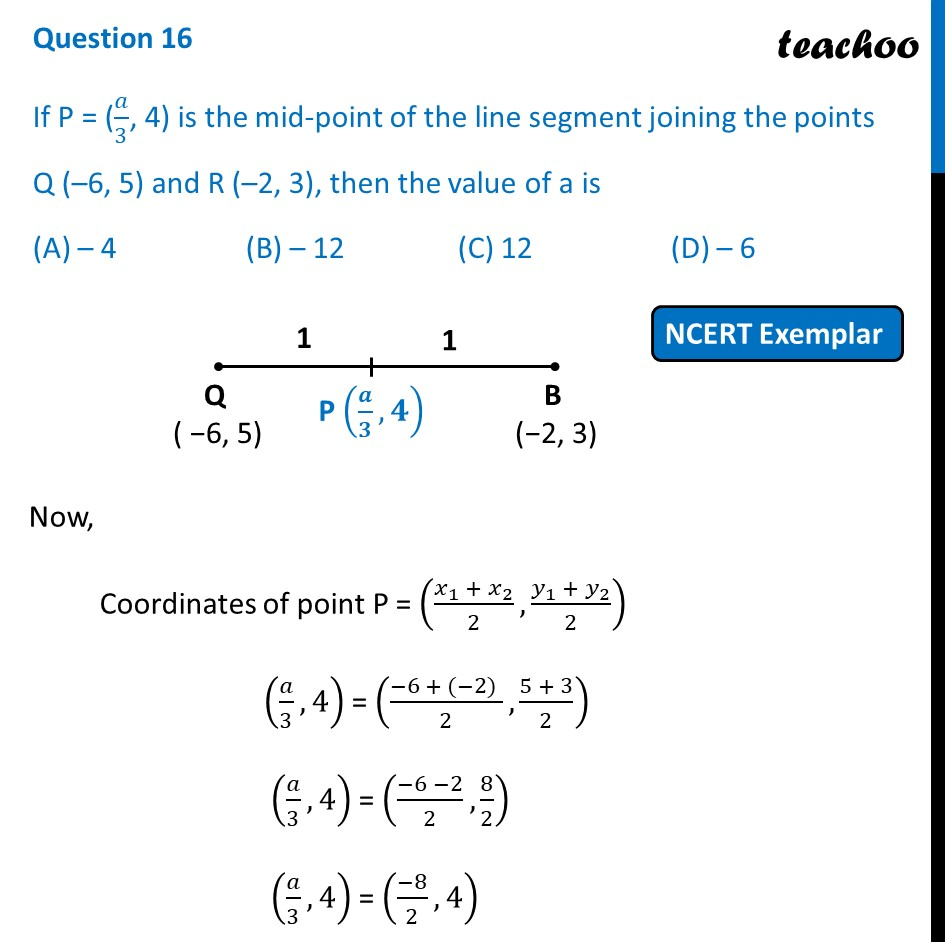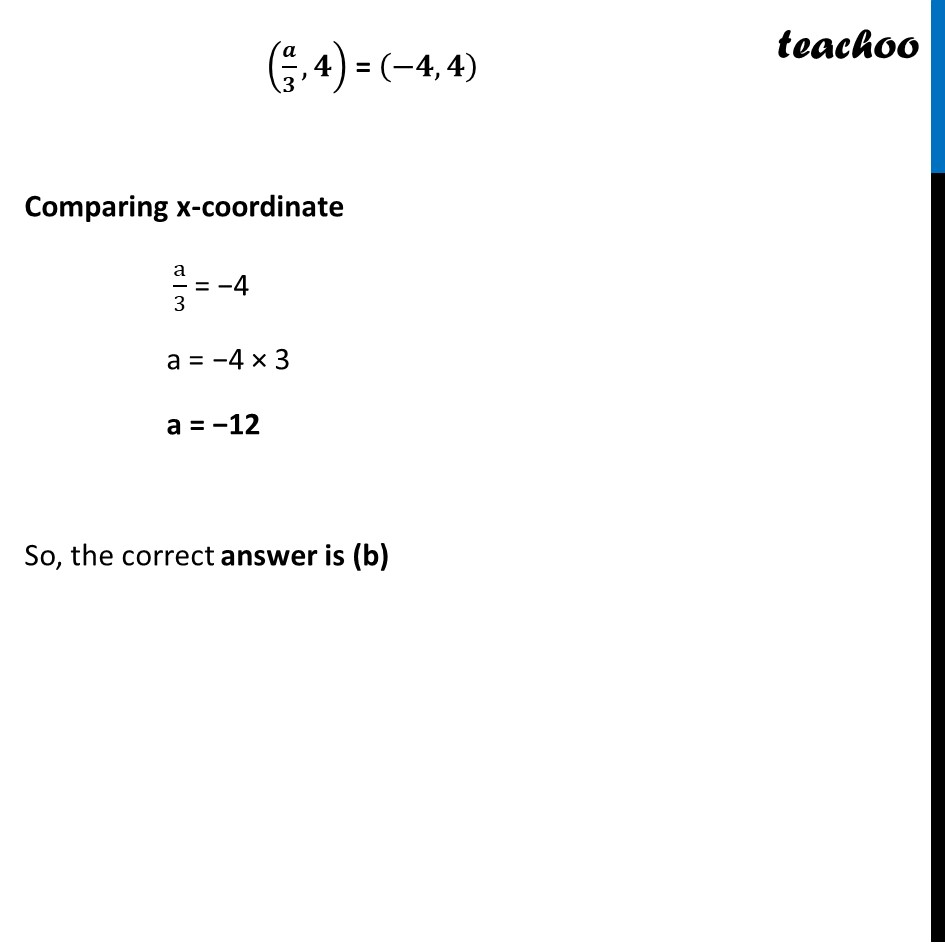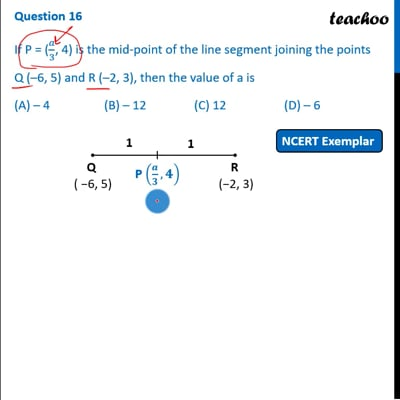NCERT Exemplar - MCQ

Chapter 7 Class 10 Coordinate Geometry
Serial order wise

## (A) – 4   (B) – 12   (C) 12   (D) – 6This video is only available for Teachoo black users

Introducing your new favourite teacher - Teachoo Black, at only ₹83 per month

### Transcript

Question 16 If P = (𝑎/3, 4) is the mid-point of the line segment joining the points Q (–6, 5) and R (–2, 3), then the value of a is (A) – 4 (B) – 12 (C) 12 (D) – 6 Now, Coordinates of point P = ((𝑥_1 + 𝑥_2)/2,(𝑦_1 + 𝑦_2)/2) (𝑎/3,4) = ((−6 + (−2) )/2,(5 + 3)/2) (𝑎/3,4) = ((−6 −2)/2,8/2) (𝑎/3,4) = ((−8)/2,4) (𝒂/𝟑,𝟒) = (−𝟒,𝟒) Comparing x-coordinate a/3 = −4 a = −4 × 3 a = −12 So, the correct answer is (b)